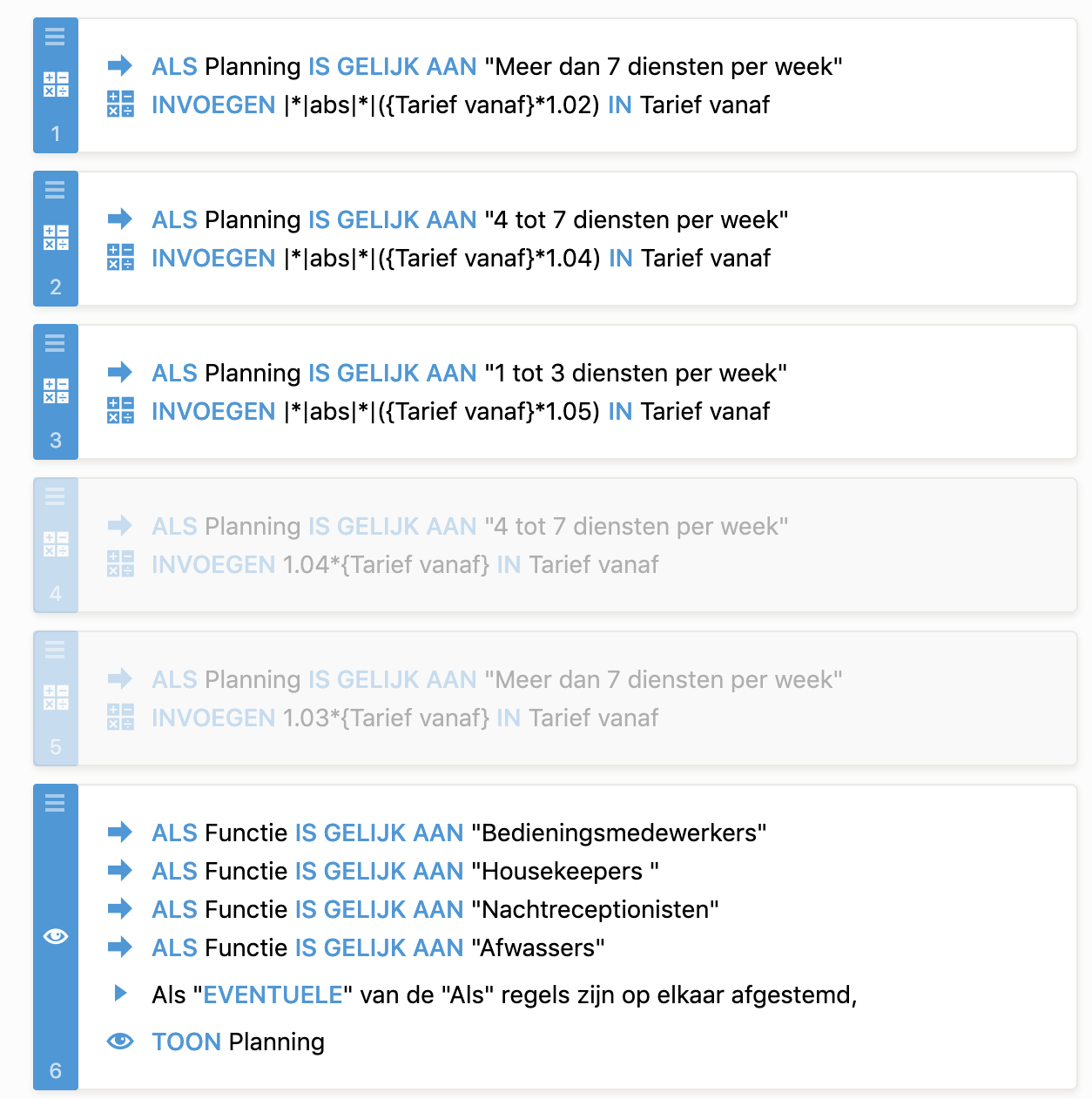# Why is my total higher than I expect by using Calculator fields?

•Recolo
Asked on April 18, 2019 at 03:59 AM

Dear support,

In the form below I am using conditional calculations. What I like to achieve is the following

field "tarief vanaf" Should multiply with factor 1,02 (2 percent increase) if the condition is met that field planning is "meer dan 7 diensten per week"

field "tarief vanaf" Should multiply with factor 1,04 (4 percent increase) if the condition is met that field planning is "4 tot 7 diensten per week"

field "tarief vanaf" Should multiply with factor 1,05 (5 percent increase) if the condition is met that field planning is "1 tot 3 diensten per week"

The outcome is a lot higher than I expect. What is not correct in the formula I am using?

Kind regards,

Koen

https://form.jotformeu.com/Recolo/tarief-calculator•gerardw
Answered on April 18, 2019 at 09:08 AM

You have used the form calculation widget and the conditional calculation with reference to the same field. I would ask you to follow the steps below and then check if your calculation becomes correct:

1. There are calculation values assigned to the dropdown fields i.e. Functie, Ervaring en leeftijd and Uren per week. Therefore I would suggest adding calculation values to the Planning field also as shown below:2. Now remove all the conditions created for the form calculation and revise the calculation in the form calculation widget as follows:After doing the above steps, now please check if you are getting the correct value as per the calculation.